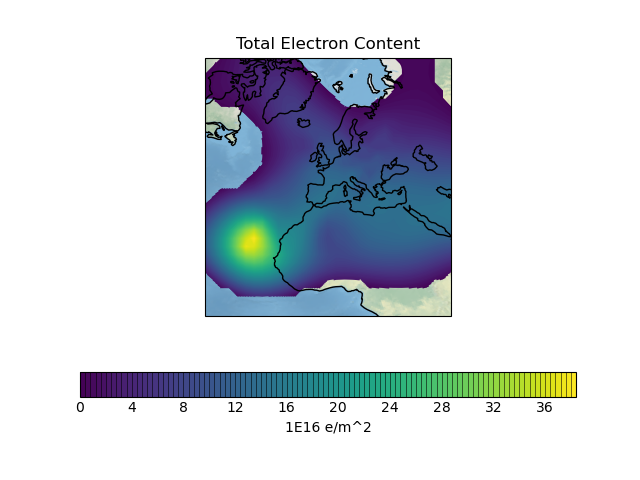# Ionosphere Space Weather#

This space weather example plots a filled contour of rotated pole point data with a shaded relief image underlay. The plot shows aggregated vertical electron content in the ionosphere.

The plot exhibits an interesting outline effect due to excluding data values below a certain threshold.```import matplotlib.pyplot as plt
import numpy.ma as ma

import iris
import iris.plot as iplt
import iris.quickplot as qplt

def main():
# Load the "total electron content" cube.
filename = iris.sample_data_path("space_weather.nc")
cube = iris.load_cube(filename, "total electron content")

# Explicitly mask negative electron content.

# Plot the cube using one hundred colour levels.
qplt.contourf(cube, 100)
plt.title("Total Electron Content")
plt.xlabel("longitude / degrees")
plt.ylabel("latitude / degrees")
plt.gca().stock_img()
plt.gca().coastlines()

iplt.show()

if __name__ == "__main__":
main()
```

Total running time of the script: ( 0 minutes 1.116 seconds)

Gallery generated by Sphinx-Gallery